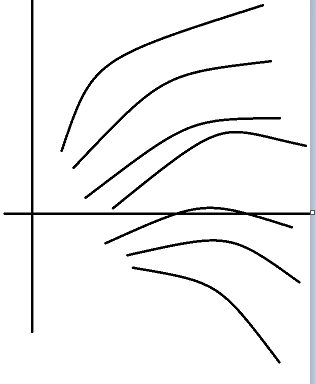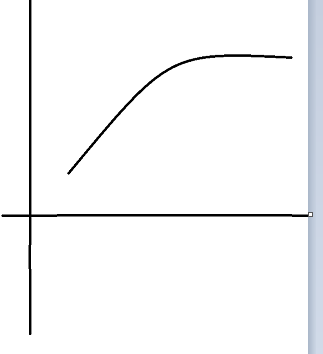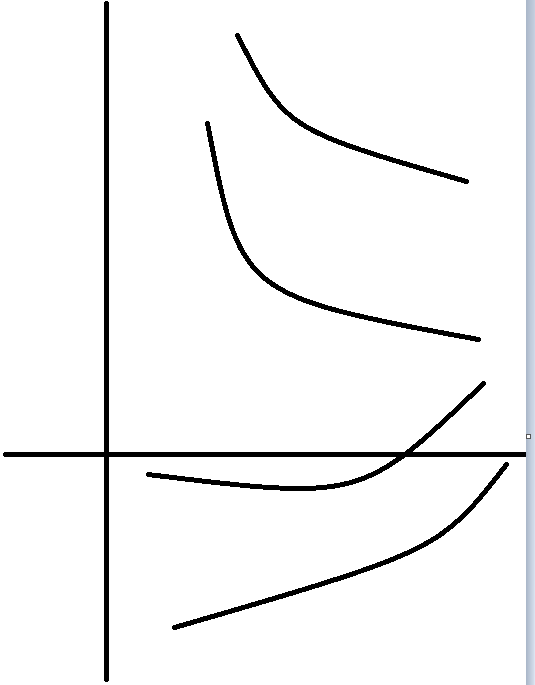# 浅谈带权二分或者斜率凸优化

APOI讲了这个东西，还有THU命题的《九省·林克卡特树》，感觉好像很热点的样子。

（(xd/yd)DP是指状态数为n^x级且每种状态的转移数为$n^y$级的DP，这个形式下的DP的最好效率是$n^{x+y}$的）

——对于某状态二元组(i,j),有最优值f[i][j]；

——每个f[i][j]的得出，都需要比较$n^y$个其他的状态二元组，从中挑出一个可以使f[i][j]更优的状态作为前驱来进行转移；

——需要某一个f[n][m]的结果（因为n和m同级，所以可以认为状态数为$n^2$级）。

F在整点处的最值，也是对于所有i而言f[n][i]的最值；

f'[b]=max(枚举c<b){f'[c]+val[c+1,b]}(每转移一次就相当于原来的f中被隐去的维度其数值+1，这意味着G的自变量+1,此时G对h'多贡献了K)$$f_{[i][j]}=MIN_{k∈[0,j-1]}f_{[i-1][k]}+(sum_{l=k+1}^{j}a[l])^2$$

$$且对于所有i>j，有f_{[i][j]}=INF$$

posted @ 2018-05-22 20:26  F.W.Nietzsche  阅读(1496)  评论(0编辑  收藏  举报